# HSSlive: Plus One & Plus Two Notes & Solutions for Kerala State Board

## इयत्ता नववी विज्ञान धडा वर्तमान वीज मराठी स्वाध्याय PDFइयत्ता नववी विज्ञान धडा वर्तमान वीज मराठी स्वाध्याय PDF

या लेखात, आम्ही विज्ञान धडा वर्तमान वीज विषयासाठी इयत्ता नववी मराठी सोल्यूशन्स देऊ. इयत्ता नववी मधील विद्यार्थी पाठ्यपुस्तकांमध्ये उपस्थित असलेल्या व्यायामांसाठी प्रश्न आणि उत्तरे डाउनलोड आणि कॉपी करण्यास सक्षम असतील.

इयत्ता नववी विज्ञान धडा वर्तमान वीजाच्या पुस्तकात महाराष्ट्र बोर्डाच्या अभ्यासक्रमातील सर्व प्रश्नांचा समावेश आहे. येथे सर्व प्रश्न पूर्ण स्पष्टीकरणासह सोडवले आहेत आणि डाउनलोड करण्यासाठी विनामूल्य उपलब्ध आहेत. महाराष्ट्र बोर्ड इयत्ता नववी विज्ञान धडा वर्तमान वीजाचे पुस्तक खाली दिले आहे. आम्‍हाला आशा आहे की आमच्‍या इयत्‍ता नववी वीच्‍या विज्ञान धडा वर्तमान वीजाचे पुस्‍तक तुमच्‍या अभ्यासात मदत करेल! जर तुम्हाला आमचे इयत्ता नववी चे पुस्तक आवडले असेल तर कृपया ही पोस्ट शेअर करा.

## इयत्ता नववी विज्ञान धडा वर्तमान वीज स्वाध्याय

 मंडळाचे नाव Maharashtra Board ग्रेडचे नाव नववी विषय विज्ञान धडा वर्तमान वीज वर्ष 2022 स्वरूप PDF/DOC प्रदाता hsslive.co.in अधिकृत संकेतस्थळ mahahsscboard.in

## समाधानासह महाराष्ट्र बोर्ड आठवा स्वाध्याय कसे डाउनलोड करायचे?

महाराष्ट्र बोर्ड नववी स्वाध्याय PDF डाउनलोड करण्यासाठी खालील स्टेप्स फॉलो करा:

1. वेबसाइट- Hsslive ला भेट द्या. 'स्वाध्याय' लिंकवर क्लिक करा.
2. महा बोर्ड नववी स्वाध्याय PDF पहा.
3. आता महाराष्ट्र बोर्ड नववी स्वाध्याय तपासा.
4. डाउनलोड करा आणि भविष्यातील संदर्भांसाठी जतन करा.

## इयत्ता नववी विज्ञान धडा वर्तमान वीज स्वाध्याय उपाय

इयत्ता नववी स्वाध्याय मधील विद्यार्थी खालील लिंक्सवरून विज्ञान धडा वर्तमान वीजाचे उपाय डाउनलोड करू शकतील.

1. The accompanying figure shows some electrical appliances connected in a circuit in a house. Answer the following questions.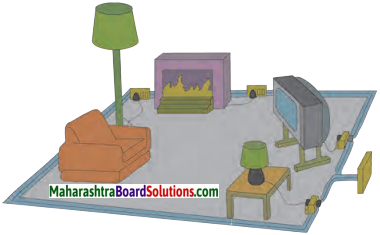A. By which method are the appliances connected?
Appliances are connected in parallel.

B. What must be the potential difference across individual appliances?
The potential difference across all appliances is same in parallel connection.

C. Will the current passing through each appliance be the same? Justify your answer.
No, as every appliance has a different load (resistance), the current flowing through each appliance will be different.

D. Why are the domestic appliances connected in this way?
The domestic appliances are connected in parallel as the potential difference remains same.

E. If the T.V. stops working, will the other appliances also stop working? Explain your answer.
No, the other devices will not stop working as the current flowing through them is along different paths.

2. The following figure shows the symbols for components used in the accompanying electrical circuit.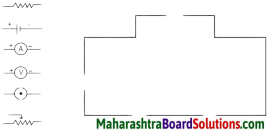Which law can you prove with the help of the above circuit?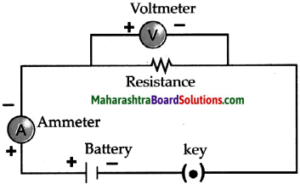(b) This circuit can be used to prove Ohm’s law.
(c) V = 1R is the expression of Ohm’s law

3. Umesh has two bulbs having resistances of 15 W and 30 W. He wants to connect them in a circuit, but if he connects them one at a time the filament gets burnt. Answer the following.

A. Which method should he use to connect the bulbs?
B. What are the characteristics of this way of connecting the bulbs depending on the answer of A above?
C. What will be the effective resistance in the above circuit?

4. The following table shows current in Amperes and potential difference in Volts.

a. Find the average resistance.
b. What will be the nature of the graph between the current and potential difference? (Do not draw a graph.)
c. Which law will the graph prove? Explain the law.

5. Match the pairs

‘A’ Group – ‘B’ Group
1. Free electrons – a. V/ R
2. Current – b. Increases the resistance in the circuit
3. Resistivity – c. Weakly attached
4. Resistances in series – d. VA/LI

6. The resistance of a conductor of length x is r. If its area of crosssection is a, what is its resistivity? What is its unit?

7. Resistances R1, R2, R3 and R4 are connected as shown in the figure. S1 and S2 are two keys. Discuss the current flowing in the circuit in the following cases.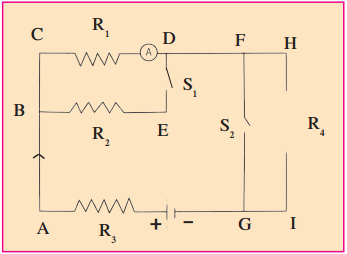a. Both S1 and S2 are closed.
b. Both S1 and S2 are open.
c. S1 is closed but S2 is open.
(a) When both S1 and S2 are dosed, the effective resistance of the circuit decreases and hence, current will increase.
(b) When both S1 and S2 are open, the effective resistance of the rircuit increases and hence, current will decrease.
(c) When S2 is closed and S2 is open, the effective resistance of the tircuit decreases and hence current will increase. [Current will be more than case (b) but less than in case (a)]

8. Three resistances x1, x2 and x3 are connected in a circuit in different ways. x is the effective resistance. The properties observed for these different ways of connecting x1, x2 and x3 are given below. Write the way in which they are connected in each case. (I-current, V-potential difference, x-effective resistance)

a. Current I flows through x1, x2 and x3
b. x is larger than x1, x2 and x3
c. x is smaller than x1, x2 and x3
d. The potential difference across x1, x2and x3 is the same
e. x = x1 + x2 + x3
f. 𝑥=11𝑥1+1𝑥2+1𝑥3

9. Solve the following problems.

A. The resistance of a 1m long nichrome wire is 6Ω. If we reduce the length of the wire to 70 cm. what will its resistance be? (Answer : 4.2Ω)
The resistance of 70cm wire will be 4.2 Ω

B. When two resistors are connected in series, their effective resistance is 80Ω. When they are connected in parallel, their effective resistance is 20Ω. What are the values of the two resistances? (Answer : 40Ω, 40Ω)
The values of the two resistances R1 and R2 are 40Ω and 40Ω.

C. If a charge of 420 C flows through a conducting wire in 5 minutes what is the value of the current? (Answer : 1.4 A)
Given: Electric charge (Q) = 420 C
Time (t) = 5 min = 5 x 60
= 300 sec.
To find: Electric current (1) = ?
Formula:
𝐼=𝑄𝑡
Solution:
𝐼=𝑄𝑡
The current in the circuit is 1.4 A.

Class 9 Science Chapter 3 Current Electricity Intext Questions and Answers

Can you recall?

Question 1.
You must have seen a waterfall. Which way does the water flow?
Water flows from a certain height of a mountain towards the ground.

Question 2.
Material: Copper and aluminium wires, glass rod, rubber.
Make connection as shown in figure 3.8. First connect a copper wire between points A and B and measure the current in the circuit. Then in place of the copper wire, connect the aluminium wire, glass rod, rubber, etc one at a time and measure the current each time. Compare the values of the current in different cases.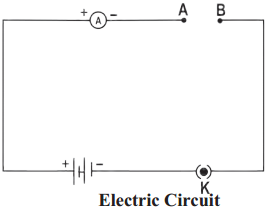Also take different metal strips (Iron, Copper, Zinc, and Aluminium) and connect it in slot AB. Now observe the difference in the resistance using Ohm meter.
When copper and aluminium wires are connected to the circuit, current flows through it, as both are good conductors of electricity. When glass rod or rubber was connected to the circuit, current does not flow through it, as both are bad conductors of electricity.

Copper displays lowest resistance while the resistance increases with aluminium, zinc and iron respectively.

Question 3.
Set up the experiment as shown in figure. Then remove the clamp from the rubber tube.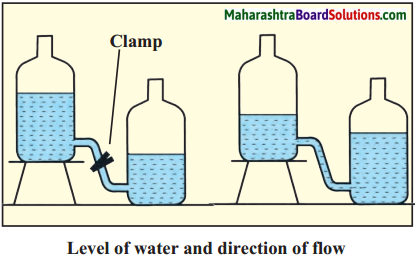(a) What happens when the clamp is removed?
When the clamp is removed, water flows from higher level to lower level.

(b) Does the water stop flowing? Why?
Yes, the water stops flowing. This happens when the level of water becomes equal in both the bottles, i.e., there is no difference in the water levels.

(c) What will you do to keep the water flowing for a longer duration?
The difference in the water level has to be maintained till that time. The difference must never be zero.

Question 4.
Point out the mistakes in the figure below: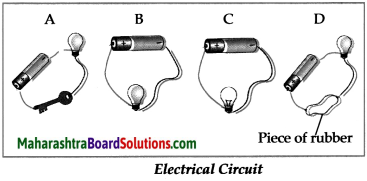A: Wire is broken at the negative terminal. Bulb will not glow as the circuit is incomplete.
B: Wire is disconnected at the negative terminal. Bulb will not glow as the circuit is incomplete.
C: The circuit is complete. Therefore, bulb will glow.
D: Rubber is a bad conductor of electricity. Hence, it will not allow current to flow and the bulb will not glow.

Question 5.
Why are the bulbs in Figures B, C and D not lighting up?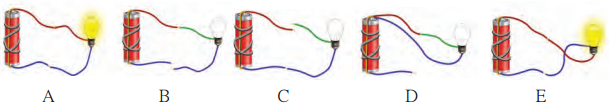• In B, the blue wire is broken. Hence circuit is incomplete and current does not flow. Therefore, bulb will not light up.
• In C, the red wire is broken. Hence circuit is incomplete and current does not flow. Therefore, bulb will not light up.
• In D, both wires are connected to the same terminal. Hence, there is no potential difference and current does not flow. Therefore, bulb will not light up.

Class 9 Science Chapter 3 Current Electricity Additional Important Questions and Answers

Choose and write the correct option:

Question 1.
1mA = …………… A.
(a) 103
(b) 10-3
(c) 106
(d) 10-6
(a) 103

Question 2.
To increase the effective resistance in a circuit the resistors are connected in ………….. .
(a) Series
(b) Parallel
(c) Both ways
(d) None of these
(a) series

Question 3.
1 kilowatt hr = …………… joules.
(a) 4.6 x 106
(b) 3.6 x 106
(c) 30.6 x 106
(d) 3.6 x 1O5
(b) 3.6 x 106

Question 4.
The voltage difference in India between the live and neutral wires is about ………….. .
(a) 110 V
(b) 220 V
(c) 440 V
(d) 60 V
(b) 220 V

Question 5.
Resistivity is the specific property of a ………….. .
(a) Area of cross-section
(b) Temperature
(c) Length
(d) Material
(d) material

Question 6.
If a P.D. of 12 V is applied across a 3Ω resistor then the current passing through it is ………….. .
(a) 36 A
(b) 4 A
(c) 0.25 A
(d) 15 A
(b) 4 A.

Question 7.
In order to measure the electric current flowing through a circuit, we connect …………… with the circuit.
(a) a voltmeter in parallel
(b) a voltmeter in series
(c) an ammeter in parallel
(d) an ammeter in series
(d) an ammeter in series

Question 8.
P and Q are two wires of same length and different cross-sectional areas and made of same material. Name the property which is same for both the wires.
(a) Resistivity
(b) Resistance
(c) Current
(d) Both (a) and (b)
(a) Resistivity

Question 9.
The following is true for identical bulbs connected in parallel.
(a) All bulbs glow with unequal brightness.
(b) If one bulb is non-functional, all will stop working.
(c) All bulbs glow with equal brightness.
(d) Bulbs function for longer time.
(c) All bulbs glow with equal brightness

Question 10.
The …………… wire is either yellow or green in colour.
(a) Live
(b) Neutral
(c) Earth
(d) Fuse
(c) earth

Question 11.
A current flows through a circuit due to the difference in …………… between two points in the conductor.
(a) Gravity
(b) Potential
(c) Resistance
(d) Fuse
(b) potential

Question 12.
…………… is the amount of charge flowing through a particular cross sectional area in unit time.
(a) Electric current
(b) Ampere
(c) Volt
(d) Force
(a) Electric current

Question 13.
The flow of …………… constitutes the electric current in a wire.
(a) Protons
(b) Neutrons
(c) Electrons
(d) Gravitons
(c) electrons

Question 14.
The conventional direction of flow of current is from …………… terminal to …………… terminal.
(a) Negative to positive
(b) Neutral to positive
(c) Positive to negative
(d) Positive to neutral
(c) positive, negative

Question 15.
Current stops flowing when potential difference between two ends of a wire becomes ………….. .
(a) Zero
(b) Positive
(c) Negative
(d) Higher
(a) zero

Question 16.
Resistances are connected in …………… so as to pass the same current through them.
(a) Series
(b) Parallel
(c) Reversed
(d) Disconnect
(a) series

Question 17.
To decrease the effective resistance in a circuit, the resistances are connected in ………….. .
(a) Series
(b) Parallel
(c) Reversed
(d) Disconnect
(b) parallel

Question 18.
1μV = …………… V
(a) 102
(b) 10-6
(c) 106
(d) 103
(b) 10-6

Question 19.
Good conductors contain a large number of ………….. .
(a) Protons
(b) Neutrons
(c) Electrons
(d) Gravitons
(c) free electrons

Question 20.
Electrons flow from …………… terminal to …………… terminal in a conductor when a potential difference is applied.
(a) Negative to positive
(b) Neutral to positive
(c) Positive to negative
(d) Positive to neutral
(a) negative, positive

Find the odd one out:

Question 1.
Voltmeter, Ammeter, Galvanometer, Thermometer
Thermometer

Question 2.
Rubber, Silver, Copper, Gold
Rubber

Question 3.
Wood, Glass, Steel, Rubber
Steel

Question 4.
Graphite, Diamond, Fullerenes, Coal
Fullerenes

Distinguish between:

Question 1.
Voltmeter and Ammeter

 Voltmeter Ammeter (i) It is an instrument used to measure the potential difference between two terminals of a cell. (i) It is an instrument to measure the electric current flowing through a circuit. (ii) It is connected in parallel with the cell. (ii) It is connected in series with the cell. (iii) It has a very high resistance. (iii) It has a very low resistance. (iv) Voltmeter has range of volts. (iv) Ammeter has range of amps.

Question 2.
Ohmic conductors and Non-Ohmic conductors

 Conductors Insulators (i) Substances which have very low electrical resistances are called conductors. (i) Substances which have extremely high electrical resistances are called Insulators. (ii) They contain a large number of free electrons. (ii) They contain practically no free electrons. (iii) Conductors are mostly metals. (iii) Insulators are mostly non metals. (iv) Conductor example iron, copper. (iv) Insulator example rubber, plastic.

Question 3.
Conductors and Insulators

 Resistance Resistivity (i) The hindrance to the flow of electrons is called resistance. (i) Resistivity is the specific property of the material of a conductor. (ii) The S.I. unit of resistance is ohm (Q). (ii) The S.I. unit of resistivity is ohm-metre (Q – m). (iii) It depends on temperature, area of cross-section, length of conductor and material of the conductor. (iii) It depends on material of the conductor. (iv) Resistance can be changed as it depends of external factor as well. (iv) Resistivity cannot be changed as it depends of internal factors.

Question 4.
Resistance in Series and Resistance in Parallel

 Resistance in Series Resistance in Parallel (i) Effective resistance of the resistors is equal to the sum of their individual resistances. (i) Inverse of the effective resistance is equal to the sum of the inverse of individual resistances. (ii) The same current flows through each resistor. (ii) The total current flowing through the circuit is the sum of the currents flowing through individual resistors. (iii) The effective resistance is larger than each of the individual resistances. (iii) The effective resistance of resistors connected in parallel is less than the least resistance of individual resistors. (iv) This arrangement is used to increase the resistance in a circuit. (iv) This arrangement is used to decrease the resistance in a circuit.

Question 5.

 Electric current Potential difference (i) The flow of electric charge per unit time is called electric current. (i) The difference in potential between the positive and negative terminal of a cell is the potential difference of that cell. (ii) The S.I. unit of electric current is ampere. (ii) The S.I. unit of potential difference volt. (iii) Ammeter is used to measure electric current. (iii) Voltmeter is used to measure electric current. (iv) Current is represented by: I=Qt (iv) Potential difference is represented by: V=WQ

Make pair:

Question 1.
Copper : Conductor :: Rubber : ……………….
Insulator

Question 2.
Aluminium : ………………. :: Indium oxide : Super Insulator
Super conductor

Question 3.
Parallel Connection : 1R𝑝=1R1+1R2 :: Series Connection : ……………….
Rs = R1 + R2

(4) Electric Current : ………………. :: Electric charge : Coulomb
Ampere

(5) Electric resistance : Ohm :: Potential difference : ……………….
Volt

State whether the following statements are true or false. Correct the false statements:

(1) The SI unit of charge is volt.
(2) Voltmeter is always connected in series with the device.
(3) The conventional direction of flow of current is from positive terminal to negative terminal.
(4) Silver and copper are good conductors.
(5) Resistivity of pure metals is more than alloys.
(6) Resistance in series arrangement is used to decrease resistance of circuit.
(7) A conducting wire offers less resistance to flow of electrons.
(8) Charges are measured in ampere.
(9) The unit of potential difference is ampere.
(10) Resistance of a conductor is inversely proportional to the length of the conductor.
(11) Ammeter is connected in parallel to the cell to measure current.
(12) Fuse is made of wire having high melting point.
(1) False. The SI unit of charge is coulomb.
(2) False. Voltmeter is ahvays connected in parallel with the device.
(3) True
(4) True
(5) False. Resistivity of pure metals is less than alloys.
(6) False. Resistance in series arrangement is used to increase resistance of circuit.
(7) True
(8) False. Charges are measured in coulomb.
(9) False. The unit of potential difference is volt.
(10) False. Resistance of a conductor is directly proportional to the length of the conductor.
(11) False. Ammeter is connected in series to the cell to measure current.
(12) False. Fuse is made of wire having low melting point.

Answer the following in one sentence:

Question 1.
Which is the unit used to measure large voltages?
Kilovolts and Megavolts are the units used to measure large voltages.

Question 2.
What is the SI unit of potential difference?
The SI unit of potential difference is volt (V).

Question 3.
What is lightning?
Lightning is the electric discharge travelling from clouds at high potential to earth’s surface which is at zero potential.

Question 4.
What is the unit of resistivity.
The unit of resistivity is ohm metre (Qm).

Question 5.
Which substances are called conductors of electricity?
Those substances which have very low electrical resistance are called conductors of electricity.

Question 6.
What is Earth wire?
Earth wire is generally yellow or green colour, it is connected to a metal plate buried deep underground near the house and is for safety purpose.

Write formula:
(1) E1ectriccurrent =𝑄𝑡
(2) Electric charge = It
(3) Potential difference = IR
(4) Electric resistance =𝑉𝐼
(5) Current =𝑉𝑅
(6) Resistivity =𝑅𝐴𝐿

Give scientific reasons:

Question 1.
Free electrons are required for conduction of electricity.

• Every atom of a metallic conductor has one or more outermost electrons which are very weakly bound to the nucleus.
• These are called free electrons. These electrons can easily move from one part of a conductor to its other parts. The negative charge of the electrons also gets transferred as a result of this motion.
• The free electrons in a conductor are the carriers of negative charge. Hence, free electrons are required for conduction of electricity.

Question 2.
Wood and glass are good insulators.

• Those substances which have infinitely high electrical resistance are called insulators.
• Wood and glass have high resistance and negligible free electrons for conduction of electricity.
• Hence, wood and glass are good insulators.

Question 3.
Connecting wires in a circuit are made of copper and aluminium.

• Copper and aluminum are good conductors of electricity.
• They have low electrical resistance and large number of free electrons.
• As they are malleable and ductile, they can be drawn into thin wires. Hence, connecting wires in a circuit are made of copper or aluminum.

Question 4.
A thick wire has a low resistance.

• The resistance (R) of a wire is inversely proportional to the cross-sectional area (A) of a wire. i.e., R i
• Thus, greater is the cross-sectional area of a conductor (wire), lower is its resistance. Hence, a thick wire has a low resistance.

Question 5.
A series combination of resistances is used to increase the resistance of a circuit.

• When resistances are connected in series, the effective resistance of the resistors is equal to the sum of their individual resistances. Rs = R1 + R2 ………….. Rn
• The effective resistance is larger than each of the individual resistances. Hence, This arrangement is used to increase the resistance in a circuit.

Question 6.
A parallel combination of resistances decreases the effective resistance of the circuit.

• In a parallel combination, the inverse of the effective resistance is equal to the sum of the inverses of individual resistances. 1Rp=1R1+1R2……⋅1Rn
• The effective resistance of resistors connected in parallel is less than the individual resistors.
• Due to this, any addition of an individual resistance in parallel combination will decrease the overall resistance of the circuit. Hence, a parallel combination of resistance decreases the effective resistance of the circuit.

Question 7.
Lightning occurs from sky to earth.

• Lightning is the electric discharge travelling from clouds at high potential to the earth’s surface, which is at zero potential.
• The earth is always at lower potential as compared to the clouds.
• Hence, lightning occurs from sky to earth.

Question 8.
In streetlights, bulbs are connected in parallel.

1. Even if any one of the several bulbs connected in parallel becomes non-functional because of some damage to its filament, the circuit does not break as the current flows through the other paths, and the rest of the bulbs light up.
2. When several bulbs are connected in parallel, they emit the same amount of light as when they are connected individually in the circuit, while bulbs connected in series emit less light than when connected individually. Hence, streetlights are connected in parallel.

Numerical:
Numericals based on the formula: (1) Q= It (2) W= VQ

Question 1.
A current of 0.4 A flows through a conductor for 5 minutes. How much charge would have passed through the conductor?
Given: Current (I) = 0.4 A
Time (t) = 5 min = 5 x 60 = 300 s
To find: Charge (Q) =?
Formula: Q = 1 x
Solution: Q = 0.4 x 300
Q= 120 C.
Charge passing through the conductor is 120

Question 2.
Find the amount of work done if 3 C of charge is moved through a potential difference of 9 V.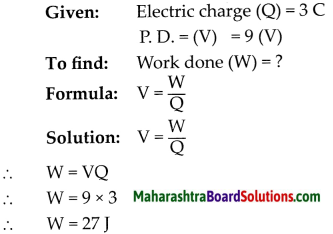The work done is 27 joule.

Question 3.
The resistance of the filament of a bulb is 1000Ω. It is drawing a current from a source of 230 V. How much current is flowing through it?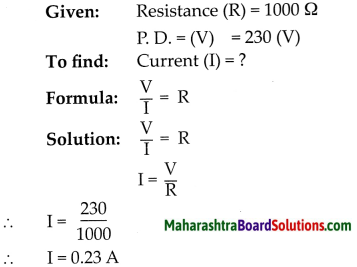The current flowing through the filament of bulb is 0.23 A.

Question 4.
The length of a conducting wire is 50 cm and its radius is 0.5 mm. If its resistance is 30Ω, what is the resistivity of its material?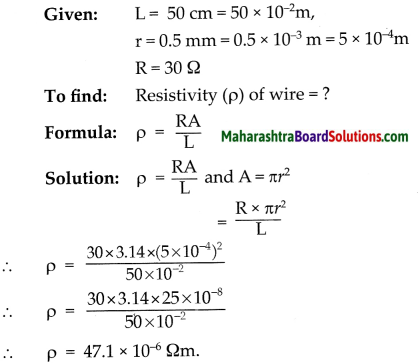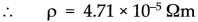The resistivity of the wire is 4.71 x 10-5 Qm.

Question 5.
A current of 0.24 A flows through a conductor when a potential difference of 24 V is applied between its two ends. What is its resistance?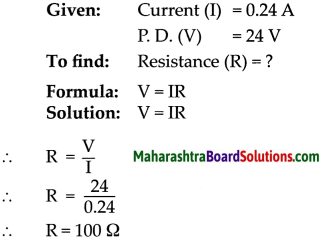The resistance of a conductor is 100Ω.

Question 6.
If three resistors 15Ω, 3Ω and 4Ω each are connected in series, what is the effective resistance in the circuit?
Given:
R1 =15Ω
R2 = 3Ω
R3 = 4Ω
Effective resistance in series (Rs) = ?
Rs = R1 + R2 + R3
Rs = 15 + 3 + 4
Rs = 22Ω
The effective resistance in the circuit is 22Ω.

Question 7.
Three resistances 15Ω, 20Ω and 10Ω are connected in parallel. Find the effective resistance of the circuit.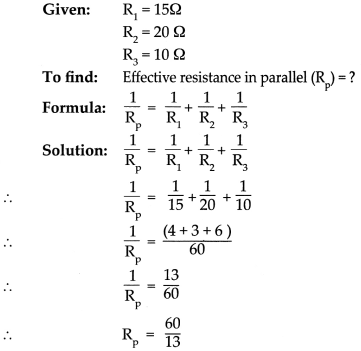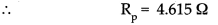The effective resistance of the circuit is 4.615 Ω. It is less than the least of the three i.e., 10Ω.

Write a note on the following:

Question 1.
Electric current
An electric current is the flow of electrons through a conductor. Quantitatively, current
(I) is defined as the charge passing through a conductor in unit time.
𝑇=𝑄𝑡

Question 2.
1 ampere
One ampere current is said to flow in a conductor if one coulomb charge flows through it every second.
1 A=1C1 s

Question 3.
1 volt
The potential difference between two points is said to be 1 volt if 1 joule of work is done in moving 1 coulomb of electric charge from one point to another.
1 V=1 J1C

Question 4.
Potential Difference
The amount of work done to carry a unit positive charge from point A to point B is called the electric potential difference between the two points.
𝑉=𝑊𝑄

Question 5.
Conductor
Those substances which have very low resistance are called conductors. Current can flow easily through such materials.

Question 6.
Insulators
Those substances which have extremely high resistance and through which current cannot flow are called insulators.

Question 7.
1 ohm
If one Ampere current flows through a conductor when one Volt potential difference is applied between its ends, then the resistance of the conductor is one Ohm.
1 Volt 1 Ampere =1Ohm

Question 8.
Potential
The level of electric charge present is known as potential.

Question 9.
Ohm’s Law
If the physical state of a conductor remains constant, the current (I) flowing through it is directly proportional to the potential difference (V) between its two ends.
V = IR

Question 10.
Superconductors
The resistance of some conductors becomes nearly zero if their temperature is decreased up to a certain value close to 0 K. Such conductors are called superconductors.

Question 11.
Non-ohmic conductors
Conductors which do not obey Ohm’s law are called non-ohmic conductors.

Complete the flow charts:

(1) Protection from Electricity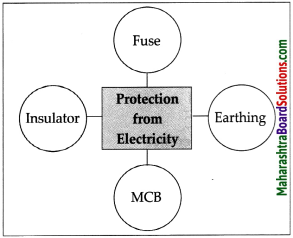(2) Resistance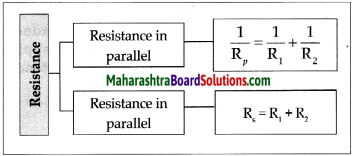(3) Resistivity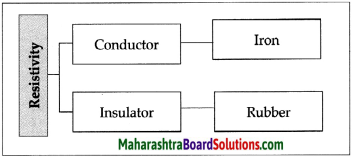Superconductors
The resistance of these conductors becomes nearly zero if their temperature is decreased up to a certain value close to 0 K. Aluminium is an example of Super Conductor. Superconductors can be used in space missions to increase/ boost the signal strength. They are also used i in the data fibres to increase the speed of data transfer.

Give explanations of the given statements:

Question 1.
Safety precautions are to be taken while using electricity.

• Electric switches and sockets should be fitted at a height at which small children cannot reach and put pins or nails inside. Plug wires should not be pulled while removing a plug from its socket.
• Before cleaning an electrical appliance it should be switched off and its plug removed from the socket.
• One’s hands should be dry while handling an electrical appliance, and, as far as possible, one should use footwear with rubber soles.
• As rubber is an insulator, it prevents the current from flowing’ through our body, thereby protecting it.
• If a person gets an electric shock, you should not touch that person. You should switch off the main switch or remove the plug from the socket if possible.
• If not, then you should use a wooden pole to push the person away from the electric wire.

Question 2.
In a domestic circuit colour code is followed while setting up electrical wiring.

• The electricity in our homes is brought through the main conducting cable either from the electric pole or from underground cables.
• Usually, there are three wires in the cable.
(a) Live wire which brings in the current. It has a red or brown insulation.
(b) Neutral wire through which the current returns. It is blue or black.
(c) Earth wire is of yellow or green colour. This is connected to a metal plate buried deep underground near the house and is for safety purposes.
• In India, the voltage difference between the live and neutral wires is about 220 V.
• Live and neutral wires are connected to the electric meter through a fuse.
• They are connected through a main switch, to all the conducting wires inside the home so as to provide electricity to every room.
• In each separate circuit, various electrical appliances are connected between the live and neutral wires.
• The different appliances are connected in parallel and the potential difference across every appliance is the same.

Question 3.
Fuse used in electrical circuit can save electrical objects from damage.

• Fuse wire is used to protect domestic appliances.
• It is made of a mixture of substances and has a specific melting point.
• It is connected in series to the electric appliances. If for some reason, the current in the circuit increases excessively, the fuse wire gets heated and melts.
• The circuit gets broken and the flow of current stops, thus protecting the appliance.
• This wire is fitted in a groove in a body of porcelain-like non-conducting material. For domestic use, fuse wires with upper limits of 1 A, 2 A, 3 A, 4 A, 5 A and 10 A are used.

Question 4.
Bulbs arranged in parallel glow brighter than bulbs arranged in series.

• The amount of light given out by bulbs in parallel combination will be more than that in series combination.
• In parallel combination the resistance of the overall circuit decreases whereas in series it increases, so the current flowing through the bulbs in parallel circuit is more.
• Due to this, intensity of light given out by bulbs in parallel combination is more than the bulbs in series combination.

Complete the following table: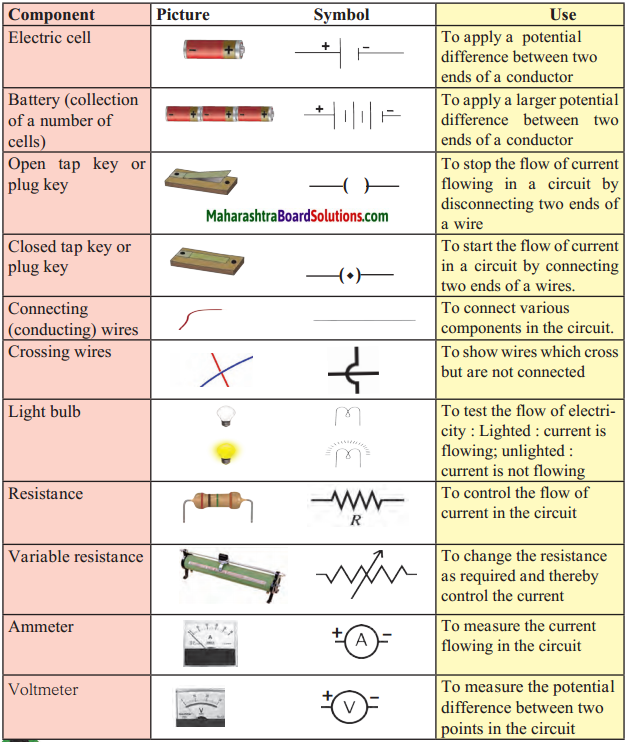Solve the numerical:

Question 1.
The length of a conducting wire is 50 cm and its radius is 0.5 mm. If its resistance is 30Ω, what is the resistivity of its material?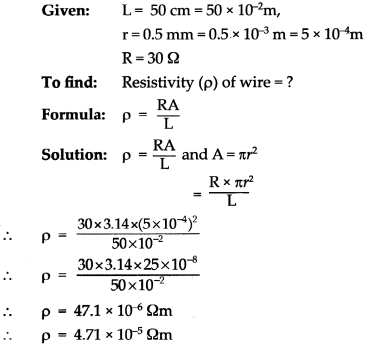The resistivity of the wire is 4.71 x 10-5 Ωm.

Question 2.
Determine the current that will flow when a potential difference of 33 V is applied between two ends of an appliance having a resistance of 110 Ω. If the same current is to flow through an appliance having a resistance of 500 Ω, how much potential difference should be applied across its two ends?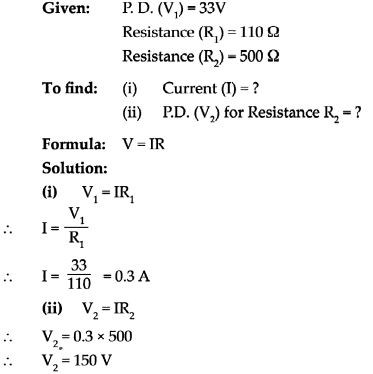The current is 0.3 A and potential difference to be applied is 150 V.

Question 3.
Determine the resistance of a copper wire having a length of 1 km and diameter of 0.5 mm.
Given: Resistivity of copper (p)
= 1.7 x 10-8 Ω m
Converting all measures into metres.
Length of wire (L) = 1 km
= 1000 m = 103 m
Diameter of wire (d) = 0.5 mm
= 0.5 x 10-3m
To find: Resistance of wire (R) = ?
Formula:
𝑅=𝜌𝐿𝐴
Solution:
If d is the diameter of the wire then, its area of cross-section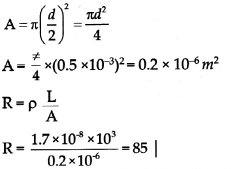The resistance of a copper wire is 85Ω and area of cross section is 0.2 x 10-6 m2

Question 5.
Two resistors having resistance of 16 and 14 are connected in series. If a potential difference of 18 V Is applied across them, calculate the current flowing through the circuit and the potential difference across each individual resistor.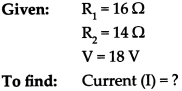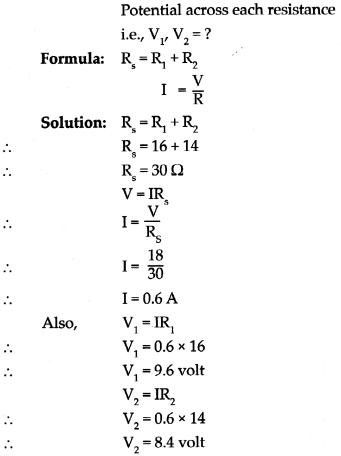The current in the circuit is 0.6 A and potential across 16 Ω retor is 9.6 volt and 14 Ω resistor is 8.4 voIt.

Question 6.
If the resistors 5 Ω, 10 Ω and 30 Ω are connected in parallel to battery of 12 V, find the effective resistance in the circuit. Calculate the total current and current in each resistor.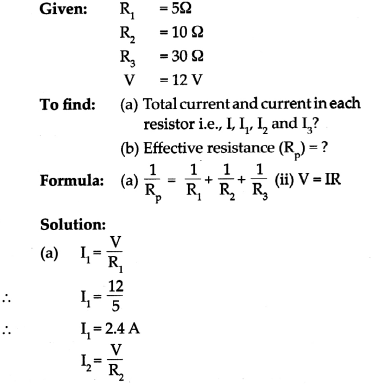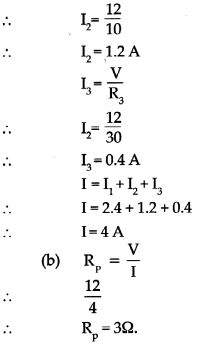(a) The total current is 4 A and current in each resistor is 2.4 A, 1.2 A and 0.4 A respectively.
(b) The effective resistance in the circuit is 3Ω.

Complete the diagram and answer the questions:

Question 1.
The following figure shows the symbols for components used in the accompanying electrical circuit.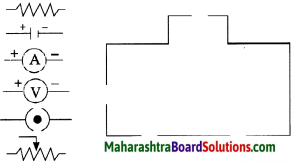(a) Place them at proper places and complete the circuit.
(b) Which law can you prove with the help of the above circuit?
(c) State expression for Ohm’s law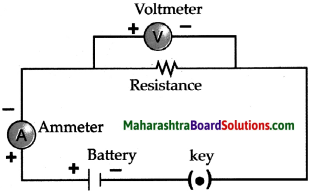(b) This circuit can be used to prove Ohm’s law.
(c) V = IR is the expression of Ohm’s law

Question 3.
Explain with the help of a diagram, what are free electrons and how they move through the conductor?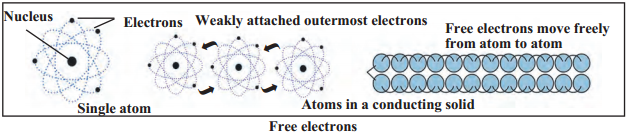• Every atom of a metallic conductor has one or more outermost electrons which are very weakly bound to nucleus.
• These are called free electrons.
• These electrons can easily move from one part of a conductor to its other parts.

Complete the paragraph:

Question 1.
If resistors are connected in series,
The same current flows through each resistor. The effective resistance of the resistors is equal to the sum of their individual resistances. The potential difference between the two extremes of the arrangement is equal to the sum of the potential differences across individual resistors. The effective resistance is larger than each of the individual resistances. This arrangement is used to increase the resistance in a circuit. This type of connection is used in electrical heating equipment like geysers, iron, and hair dryers.

Question 2.
If a number of resistors are connected in parallel,
The inverse of the effective resistance is equal to the sum of the inverses of individual resistances. The current flowing through an individual resistor is proportional to the r inverse of its resistance and the total current flowing through the circuit is the sum of the currents flowing through individual resistors. The potential difference across all r resistors is the same. The effective resistance of resistors connected in parallel is less than r the least resistance of individual resistors.

This arrangement is used to reduce the resistance in a circuit. Even if any one of the several bulbs connected in parallel becomes non-functional because of some damage to its filament, the circuit does not break as the current flows, through the other paths, and the rest of the bulbs light up. When several bulbs are connected in parallel, they emit the same amount of light as when they are connected individually in the circuit, while bulbs connected in series emit less light than when connected individually.

Electric switches and sockets should be fitted at a height at which small children cannot reach and put pins or nails inside. Plug wires should not be pulled while removing a plug from its socket. Before cleaning an electrical appliance it should be switched off and its plug removed from the socket. One’s hands should be dry while handling an electrical appliance, and, as far as possible, one should use footwear with rubber soles. As rubber is an insulator, it prevents | the current from flowing through our body, thereby protecting it. If a person gets an electric shock, you should not touch that person. You should switch off the main switch and if the switch is too far or you do not know where it is located, then you should remove the plug from the socket if possible. If not, then you should use a wooden pole to push the person away from the electric wire.

(i) Why should the electrical sockets be fitted at a certain height?
Electric switches and sockets should be fitted at a height at which small children cannot reach and put pins or nails inside.

(ii) Why plug wires should not be pulled out while removing any electrical device?
Plug wires should not be pulled out while removing any electrical device as it may cause the wire to break causing short circuit which can lead to fire or death.

(iii) Why should a person wear footwear with rubber soles while handling electrical appliances. .
As rubber is an insulator, it prevents the current from flowing through our body, thereby protecting it. Hence a person should wear footwear with rubber soles while handling electrical appliances.

(iv) Saee is touching an electrical button socket with wet hands what will you advise her and why?
We will advise her to dry her hands before touching any electrical sockets or devices as water on the hands can cause an electrical short circuit producing shock to the person touching it.

(v) Sneha is getting an electrical shock what will you do the save her life?
We should switch off the main switch and if the switch is too far or we do not know where it is located, then we should remove the plug from the socket if possible. If not, then we should use a wooden pole to push the person away from the electric wire.

(vi) Give a title to the above passage.
Precautions to be taken while using electricity

Question 1.
Find the expression (i.e., derive the expression) for the resistors connected in series.
Expression for the resistance connected in series:
(i) Let R1, R2 and R3 be three resistances connected in series between C and D.
(ii) Let Rs be the effective resistance in circuit and V1, V2 and V3 be the potential difference across R1, R2 and R3 respectively.
(iii) Let the potential difference across CD be V.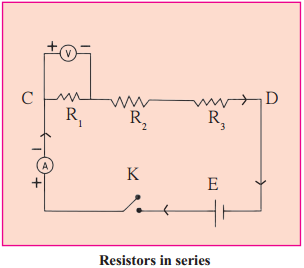(iv) In series combination.
v = v1 + v2 + v3 ……………………(i)
By using Ohm’s law
V = IRs
∴ V1= IR1, V= IRand V= 1R3
Substituting these values in equation (j) we get
IR= IR+ IR+ IR3
∴ Rs = R1 + R2 + R3
For ‘n’ number of resistors conneded in series we get
Rs = R1 + R2 + R+ …………………. + Rn

Question 2.
Find the expression (i.e., derive the expression) for the resistors connected in parallel.
Expression for the resistance connected in parallel.
(i) Let R1, R2 and R3 be the three resistances connected in parallel combination between points C and D and let R be their effective resistance.
(ii) Let I1, I2 and I3 be the currents flowing through resistances R1, R2 and R3 respectively. Let I be the current flowing through the circuit and V be the potential difference of the cell.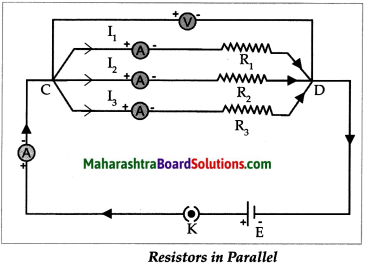(iii) For parallel combination of resistances,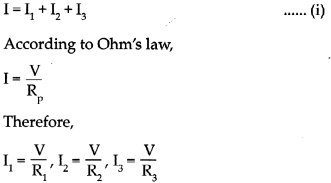(iv) Substituting the values of (I, I1, I2 and I3) in equation (i) we get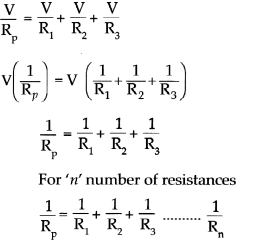Question 3.
Find the expression for resistivity of a material.
(i) At a given temperature, the resistance (R) of a conductor depends on its length (L), area of cross-section (A) and the material it is made of. If the resistance of a conductor is R, then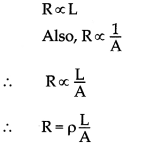(ii) p is the constant of proportionality and is called the resistivity of the material.
(iii) The unit of resistivity in SI units is Ohm metre (Ω m).
(iv) Resistivity is a specific property of a material and different materials have different resistivity. ’

Make the concept diagram and explain:

Question 1.
Make the concept diagram of an electrical circuit and explain the working of a fuse.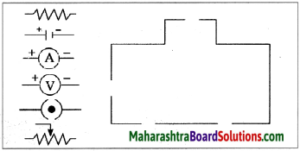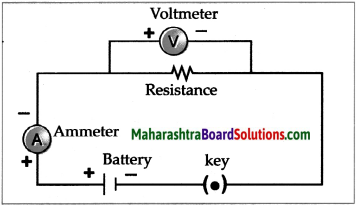• Fuse wire is used to protect domestic appliances.
• It is made of a mixture of substances and has a specific melting point.
• It is connected in series to the electric appliances. If for some reason, the current in the circuit increases excessively, the fuse wire gets heated and melts. The circuit gets broken and the flow of current stops, thus protecting the appliance.
• This wire is fitted in a groove in a body of porcelain-like non-conducting material. For domestic use, fuse wires with upper limits of 1A, 2A, 3A, 4A, 5A, and lO Aareused.

(2) Show motion of electrons in an circuit and explain precautions while using an electrical device.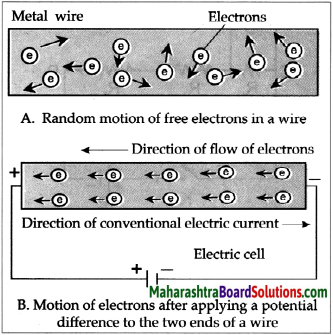• Electric switches and sockets should be fitted at a height at which small children cannot reach and put pins or nails inside. Plug wires should not be pulled while removing a plug from its socket.
• Before cleaning an electrical appliance it should be switched off and its plug removed from the socket.
• One’s hands should be dry while handling an electrical appliance, and, as far as possible, one should use footwear with rubber soles.
• As rubber is an insulator, it prevents the current from flowing through our body, thereby protecting it.
• If a person gets an electric shock, you should not touch that person. You should switch off the main switch or remove the plug from the socket if possible.
• 1f not, then you should use a wooden pole to push the person away from the electric wire.

Q.4.4.Complete the incomplete figure and give an explanation: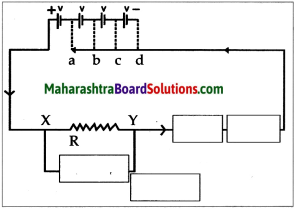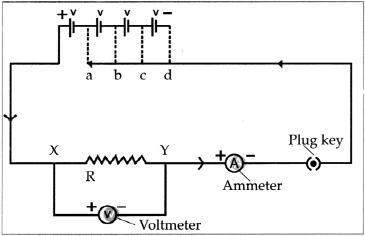If the physical state of a conductor remains constant, the current (I) flowing through it is directly proportional to the potential difference (V) between its two ends.

I α V
I = kV (k = constant of proportionality)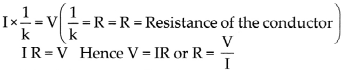This is known as Ohm’s law.
We can obtain the SI unit of resistance from the above formula, Potential difference and current are measured in Volts and Amperes respectively. The unit o resistance is called Ohm. It is indicated by the symbol Ω.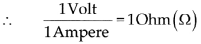The resistance of one Ohm : If one Ampere current flows through a conductor when one Volt potential difference is applied between its ends, then the resistance of the conductor is one Ohm.

## निष्कर्ष

आम्हाला आशा आहे की महाराष्ट्रावरील हा लेख तुम्हाला 'नववी विज्ञान धडा वर्तमान वीज स्वाध्याय' मदत करेल. तुम्हाला काही प्रश्न असल्यास, त्यांना खाली टिप्पणी विभागात मोकळ्या मनाने पोस्ट करा. आम्ही लवकरात लवकर तुमच्याकडे परत येऊ.
Share: